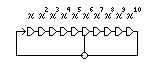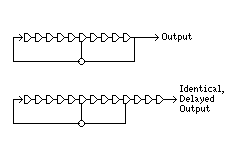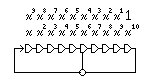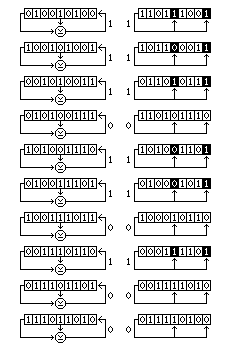[Next] [Up/Previous] [Index]

# Shift-Register Stream Ciphers

The linear feedback shift register, most often used in hardware designs, is the basis of the stream ciphers we will examine here. A string of bits is stored in a string of memory cells, and a clock pulse can advance the bits one space in that string. The XOR of certain positions in the string is used to produce the new bit in the string for each clock pulse. It is possible to choose the positions in the string to XOR so that, as long as the memory cells are not initially loaded with all zero bits, the period of the sequence of bits produced by that XOR is 2^n-1, where n is the number of cells in the string.

The following diagram illustrates an LFSR associated with the polynomial

```x^10 + x^6 + 1
``````      ---------------------------------------------------------------------
|      |      |      |      |      |      |      |      |      |      |
-->| x    | x^2  | x^3  | x^4  | x^5  | x^6  | x^7  | x^8  | x^9  | x^10 |
|   |      |      |      |      |      |      |      |      |      |      |
|    ---------------------------------------------------------------------
|                                         |                           |
----------------------------------------XOR--------------------------
```

The x^10 and x^6 terms in the polynomial correspond to the two tapped cells in the shift register shown; the 1 in the polynomial does not correspond to a tap. If the polynomial to which an LFSR corresponds is primitive, which means that in addition to being irreducible (a property similar to the property of being prime for an integer) it satisfies some additional mathematical conditions, the LFSR will have its maximum possible period, which is (2^n)-1 where n is the length of the shift register in cells. Because an LFSR works by taking the XOR of selected bits in its internal state, any LFSR containing all zero bits will never move to any other state, and so that one possible state must be excluded from any cycle of more than one state.

An LFSR with maximum period always has an even number of taps. Also, the cell with the oldest bit in the shift register is always tapped. This rule is very general, and applies even to nonlinear shift registers, for a simple reason that can be seen in the following diagram:the sequence produced from a shift register whose last few cells are not tapped is identical to that produced by a shift register otherwise identical from which those cells are omitted, except for a delay.

(Note that some popular references on cryptography erroneously show the x^n term in an LFSR's characteristic polynomial, which is always present, as corresponding to the cell with the newest bits, and show the x term, which is not always present, as corresponding to the cell with the oldest bits, which in fact must always be tapped. This is a result of diagrams that show the bits in the LFSR as moving in the wrong way.)

It should be noted, however, that primitive polynomials have a reversal property that does allow an alternative way of matching the terms in a polynomial to the possible taps in a shift register, as illustrated below:but in the reverse case, the cell with the oldest bits corresponds to the always-present 1 term (thus, the equivalents only go up to x^(n-1), and x^n does not correspond to a possible tap), not to the x term, which need not be present in the polynomial.

The reversal property is this: the polynomial

```x^n + x^p + x^q + x^r + ... + 1
```

is primitive if and only if the polynomial

```x^n + ... + x^(n-r) + x^(n-q) + x^(n-p) + 1
```

is also primitive.

### Well-known Polynomials

Incidentally, just as it is unwise to use a very well-known word or phrase as a key, some primitive polynomials modulo 2 are also "well-known", since they have been used in common systems.

Some examples are:

Used in Cyclic Redundancy Check codes:

```             12  11  3
CRC-12:     x  +x  +x +x+1

16  15  2
CRC-16:     x  +x  +x +1

16  12  5
CCITT:      x  +x  +x +1

32  26  23  22  16  12  11  10  8  7  5  4  2
AUTODIN II: x  +x  +x  +x  +x  +x  +x  +x  +x +x +x +x +x +x+1
```

Permitted (under regulations which are now out of date) for use in generating spread-spectrum sequences by radio amateurs:

```             7
7-bit:     x +x+1

13  4  3
13-bit:     x  +x +x +x+1

19  5  2
19-bit:     x  +x +x +x+1
```

Originally alleged as used in the A5 European cellular telephone algorithm:

```             19  5   2
x  +x  +x +x+1

22  9  5
x  +x +x +x+1

23  4  3
x  +x +x +x+1
```

Actually used in the A5 cellular telephone algorithm, according to more recent information:

```             19  5   2
x  +x  +x +x+1

22
x  +x+1

23  15  2
x  +x  +x  +x+1

17  5
x  +x  +1
```

Used by GPS satellites:

```             10  3
x  +x +1

10  9  8  6  3  2
x  +x +x +x +x +x +1
```

Note that the A5 polynomials (in the older version, at least) are used with the x^n-1 to 1 convention, and feedback is concentrated on the older bits in the shift register. (This, and not the x^n to x convention, going the other way, may well be the usual one.)

Also, while all LFSRs, used directly, are insecure, LFSRs with many taps (not having "sparse" feedback polynomials) produce a sequence that seems more random, and when used in a system that combines several LFSRs, combined in a way that achieves cryptosecurity, they are better. Presumably, that feature is also useful when LFSRs are used in performing CRCs; note especially the AUTODIN II (AUTODIN is a U.S. military communications system) polynomial above.

### The Galois Configuration

In addition to the conventional configuration, where each new bit input to the shift register is the XOR of several bits in the register, a shift register may also be implemented in Galois configuration, where the single bit shifted out of the register is XORed with several bits in the shift register.

The following diagram illustrates the relation between the conventional configuration and the Galois configuration of a shift register:In the conventional configuration, the bits moving through the shift register are also bits in the sequence it generates as output. Hence, each new bit entered into the register is the XOR of several previous bits in the sequence.

In the Galois configuration, generated bits are also the XOR of previous bits in the sequence, but in this case as the oldest bit included in that XOR moves through the shift register, it is XORed one at a time with the other bits as it reaches the appropriate positions in the shift register.

### The Galois Field

Speaking of Evariste Galois, the reason that primitive polynomials modulo 2 are important is that by using them as the modular polynomial in polynomial multiplication, one can create a Galois Field of order 2^n with a polynomial beginning with x^n.

A field is an algebra with both addition and multiplication, the elements of which form a group under the addition operation, and in which the multiplication operation, over all the elements of the field except zero, also creates a group. Thus, addition and multiplication modulo a prime create a finite field.

The term Galois Field is used to refer to finite fields, because Galois proved that the only finite fields were either those whose order was a prime, and were of the type described above, or those whose order was a power of a prime, and whose elements were treated as polynomials, the coefficients of which were modulo that prime, the polynomials themselves being modulo a modular polynomial which was not merely irreducible (not factorable into smaller polynomials) but also primitive; the kind of polynomial that can produce a shift register.

Here is one representation of the Galois Field of order 8 (or 2^3):

``` +   0 1 2 3 4 5 6 7   *   0 1 2 3 4 5 6 7
----------------      ----------------
0 | 0 1 2 3 4 5 6 7   0 | 0 0 0 0 0 0 0 0
1 | 1 0 3 2 5 4 7 6   1 | 0 1 2 3 4 5 6 7
2 | 2 3 0 1 6 7 4 5   2 | 0 2 4 6 3 1 7 5
3 | 3 2 1 0 7 6 5 4   3 | 0 3 6 5 7 4 1 2
4 | 4 5 6 7 0 1 2 3   4 | 0 4 3 7 6 2 5 1
5 | 5 4 7 6 1 0 3 2   5 | 0 5 1 4 2 7 3 6
6 | 6 7 4 5 2 3 0 1   6 | 0 6 7 1 5 3 2 4
7 | 7 6 5 4 3 2 1 0   7 | 0 7 5 2 1 6 4 3
```

This particular representation of that field is the one which uses x^3+x+1 as the modular polynomial. Thus, the bits in the binary representation of the numbers in these tables are treated as the coefficients of polynomials. Since polynomial addition deals with each coefficient independently, and it is done here modulo 2, the addition table should look familiar: it is the table for the bitwise XOR operation.

The field illustrated can be denoted as GF(2^3) or GF(8). The group which multiplication, exclusive of zero, forms is isomorphic to addition modulo 7; that is, it is the cyclic group of order 7. In general, this is true for any Galois field; for GF(n), the multiplicative group is the cyclic group of order n-1. While the table looks different, this means that it can be made identical to that for addition modulo 7 by replacing each of the numbers from 1 to 7 by another number from 0 to 6.

There is further discussion of Galois Fields in the description of the Decorrelated Fast Cipher, the description of Rijndael, and on a page specifically about that topic.

### Finding Maximum-Period LFSRs

We have seen above how to construct a shift register from its corresponding polynomial. And it is noted that the polynomial must be primitive for the shift register to have maximum period.

How does one construct primitive polynomials?

One way is to construct arbitrary polynomials which correspond to the properties of the shift register sought, in length and in number of taps, and test them. This, at least, can be done fairly simply.

If the polynomial is of the form x^n + ... + 1, that is, of degree n, then (for a polynomial whose coefficients are modulo 2) the condition that must be met is that x^(2^n-1) must be equal to 1 modulo the polynomial, but x^(2^n-1)/p for any prime p which divides 2^n-1 must not be equal to 1 modulo the polynomial.

If the coefficients of the polynomial were modulo 3, we would use 3^n-1, and so on.

Let us take the polynomial x^7+x+1. This corresponds to the binary string 10000011. 2^7-1 is 127, and is the maximum period of a shift register built from a polynomial of degree 7. x^127 modulo x^7+x+1 is what the binary string consisting of a 1 followed by 127 zeroes would become, after being XORed with 10000011 shifted as far left as necessary to zero out its first digit, repeated until one obtains a 7-bit string. Doing that is exactly equivalent to running the shift register in the Galois configuration, and so the reason for the condition is now obvious: if the shift register has maximum period, the 0000001 state will recur at the end of that period; and if it does so recur, this recurrence mustn't be a repeated occurrence. The only possibilities to eliminate to exclude that are the periods of which the maximum period is a multiple, and dividing the maximum period by its prime factors alone eliminates them all: the state 0000001 might recur more often, but it won't miss having one of those numbers as a multiple of the period.

Of course, applying 10000011 to 1 followed by 127 zeroes would be somewhat slow, and for a much longer shift register, 2^n-1 would be an enormous number. However, finding x^n by polynomial multiplication can be done by repeated squaring, just as that technique can be used to speed up taking numbers to powers, it can be used for exponentiation in other domains as well.

When n is composite, 2^n-1 has some obvious factors. That's because 111111 is equal to 111 times 1001, and it's also equal to 11 times 10101. That's as true in binary notation as in decimal notation. This doesn't mean that there aren't other factors of 2^n-1, though. Thus, when n is prime, 2^n-1 is not always prime. Sometimes it is, though, and then 2^n-1 is called a Mersenne prime. If the degree of the polynomial to be tested is a value of n for which 2^n-1 is a Mersenne prime, it is simpler to test if the shift register polynomial is primitive. However, doing the extra tests is not too great a problem; it's factoring 2^n-1 which, in general, might be the difficult step.

### Using LFSRs to Build Secure Ciphers

The output from an LFSR is often strengthened by using another LFSR to control how often it is stepped. This technique can be applied in the same ways that were used with pinwheels in the chapter on telecipher devices. Another technique, the Geffe generator, uses three LFSRs with different periods. One is used to choose which of the two other ones has its output used. Although a simple Geffe generator is still not secure, more elaborate constructions using that principle may be effective.

Here is a diagram showing one such construction:

``` --------
|LFSR 1|----------------------------
--------                           |
|
--------                           |
|LFSR 2|----------------------     |
--------                     |     |
|     |
--------                     |     |
|LFSR 3|----------------     |     |
--------               |     |     |
|     |     |
--------               |     |     |
|LFSR 4|----------     |     |     |
--------         |     |     |     |
---   ---    |     |
--------        \  \ /  /    |     |
|LFSR 5|-------->|     |     |     |
--------        /  / \  \    |     |
---   ---   ---   ---
|     |    \  \ /  /
|     ----->|     |
|          /  / \  \
|          ---   ---
|                 |
|                 |
--------------->(XOR)
|
--- output
```

The generator pictured uses five LFSRs. One is used to choose which of two other LFSRs is used for two purposes (each one is used for one of those purposes, the bit only swaps them):

• choosing which of two other LFSRs is used to contribute to the output, and
• being XORed with the output of the chosen LFSR.

If only four shift registers are used, so that we XOR the output of one shift register with the output of a Geffe generator, then we still have the same weakness that the Geffe generator alone had. That is because the XOR of two LFSRs, by itself, is a linear construct, and thus is as vulnerable to attack as an LFSR of the length of the two combined. So, since the one Geffe generator means that the first LFSR is XORed to one of two others, we have two alternating possibilities, both weak, which the output bitstream matches 75% of the time.

The design as given, however, provides the XOR of two shift registers, both of which can be either of two possibilities. So would the XOR of the outputs of two Geffe generators.

The design above has the advantage of requiring one fewer input. Is it secure? As noted above, the XOR of two LFSRs is no more secure than the output of a single longer LFSR. The output of this construct will agree with the XOR of LFSR 1 and LFSR 4, for example, 62.5% of the time, since 25% of the time those two LFSRs will be selected as the inputs to the final LFSR, and the other 75% of the time, other inputs will be selected, with a 50% probability of coincidentally matching that result, in the same way as, in a Geffe generator, 50% of the time the correct LFSR is used, and 25% of the time, the wrong LFSR agrees with the right one. So there is still an output bit stream which is biased towards matching an easily solved bit stream, but at least the bias is fairly weak.As a nonlinear function of the bits in an LFSR can produce any series of output bits produced by any other device with the same period (proof: use all the bits in the LFSR's state as an address to find a bit in a ROM containing the desired sequence in a suitable order), and an LFSR has a maximum period of 2^n-1, which is only one less than that of any other device with n bits of internal state, it seems it is not necessary to pursue developing the theory of nonlinear feedback shift registers with known period.

Thus, one way of using a shift register to create a series of pseudorandom bits is to take some of its bits, and use circuitry to produce a nonlinear function of them, as illustrated at right.

[Next] [Up/Previous] [Index]